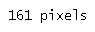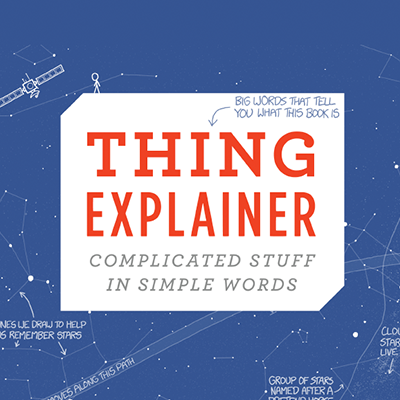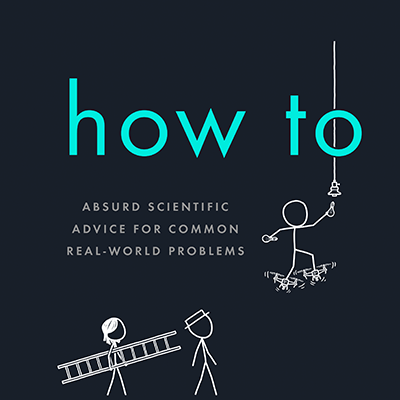serious answers to absurd questions and absurd advice for common concerns from xkcd's Randall Munroe

#### the news

What If? 2: Additional Serious Scientific Answers to Absurd Hypothetical Questions is out! Order here!## Ink Molecules

Suppose you were to print, in 12 point text, the numeral 1 using a common cheap ink-jet printer. How many molecules of the ink would be used? At what numerical value would the number printed approximately equal the number of ink molecules used?

David Pelkey

This is the kind of problem where Fermi estimation comes in handy. In Fermi estimation, we're not concerned about exact numbers. We just want, before we start doing research, to get an idea of how big the number is going to be. Will it have 10 digits, or 100 digits, or a zillion?

We'll see what we can figure out before we look anything up.

An inkjet cartridge lets me print out some number of 8.5"x11" black-and-white pages. Let's be optimistic and say a few hundred. If each page has 500 words and each word has 5 letters, then each page has 2,500 letters. 100 pages is 250,000 letters and 400 would be 1,000,000. So the number of letters per cartridge probably has six digits.

Now, how many molecules are in an ink cartridge? This will be harder to estimate without cheating and looking things up, but let's try.

Let's say I remember hearing about "Avogadro's number" in chemistry class, but I don't remember exactly what it is. It's definitely something times 1023, so it has 24 digits. And I remember that it's the number of atoms in some number of grams of something. It was a smallish number. Probably.For the record, it's 6.022×1023, and it's the number of carbon-12 atoms in 12 grams of carbon-12 (or the number of hydrogen atoms in a gram of hydrogen).Inkjet cartriges probably also contain a small number of grams of ink.Citation: If it were a big number, they would be hard to pick up, and if it were less than a gram, the idea that we've been paying $30 for them is just too upsetting to contemplate. Let's assume it's the same small number, because Fermi estimation lets us do that. I have no idea what's in ink. (Remember, we're not allowed to look stuff up yet.) I know squid can make ink of some kind, so maybe ink has some big complicated organic molecules in it. That's bad, because I have no chance of estimating their weights to within even a few orders of magnitude. Fortunately, what we need to worry the most about is the smallest molecules, because they'll contribute the most to the total count. Ink probably has a lot of water in it, like many liquids. On the other hand, I bet most of those water molecules wander off when the ink dries—since that's what the word "dries" means.We have nothing to go on here, so let's take a wild guess and suppose that a 10% of ink's bulk comes from large numbers of little molecules, ones comparable in size to the [mumble mumble carbon or something] atoms in Avogadro's number. Since Avogadro's number has 24 digits, 10% of it would be a 23-digit number. If our other guesses are right, then the number of molecules in an ink cartridge might also have about 23 digits If there are a 23-digit number of molecules in an ink cartridge, and that cartridge prints a 6-digit number of letters, then each printed letter (or number) should contain a number of ink molecules with 23 - 6 = 17 digits.What we're doing here is dividing by subtracting the number of digits. If you think this is a cool shortcut, and decide to develop it further and make it a little more rigorous and precise, then congratulations! You've just invented logarithms. That means a printed 10-digit number contains about an 18-digit number of ink molecules, and a 100-digit number contains a 19-digit number of ink molecules. Aha! The crossover point, where the number of molecules and the printed number are equal, must happen somewhere between 18 and 19 digits. So our answer, according to Fermi estimation, is in the neighborhood of a high 18-digit number. We might be off by several orders of magnitude in either direction, but in either case, it's definitely a number you could print out on a single line.Now, let's do some actual research and find out how we did. Inks, unsurprisingly, are complicated and vary a lot. Color inks contain a lot of large and heavy molecules, especially some of the pigments. Fortunately, cheap black inks—which are what David asked about—are simpler. As our example, we'll take the ink used in the random HP printer at my house. HP doesn't disclose everything about what the ink is made of, but they do publish a material safety data sheet for it here. The MSDS data tells us that the ink is over 70% water. It also contains the molecule 2-pyrrolidone (which is apparently used to synthesize the anti-seizure drug Ethosuximide) and 1,5-pentanediol. In addition, it contains up to 5% "modified carbon black", a form of crystalline carbon (like graphite and diamond). This is great news for our estimate, because crystalline carbon is very simple; its molecular formula is just "C".Assuming you count each carbon atom separately. You could interpret David's question to mean particles of ink, so each hunk of carbon black would only count as 1. However, that would mean working out exactly what water fraction remains in the dried ink and how much weight 1,5-pentanediol contributes and so forth, and that sounds like more work. Conveniently, "C" is also what's used in the definition of Avogadro's number. Small consumer cartridges contain a few grams of ink, which is less than the 12 grams used in Avogadro's number. That might make our estimate about half a digit too high. And while we were lucky at guessing carbon, HP ink contains less than 5% carbon black, not the 10% we guessed. That pushes the real answer down even lower than our estimate. But all in all, we did pretty well! Of course, this is a reminder of how much easier the digital world is:It's also a reminder of how expensive ink is. Speaking of which, the ink sac from the tiny Octopoteuthis deletron squid are probably a few milliliters, based on the collection bottle sizes mentioned in this paper, for a squid that probably only weighs a hundred grams or so.$30 could probably get you a few kilograms of fresh whole squid, and—if you picked the right squid—a total of five or six cartridges worth of ink.Lifehacks.

#### the books##### What If?

Serious Scientific Answers to Absurd Hypothetical Questions##### Thing Explainer

Complicated Stuff in Simple Words##### How To

Absurd Scientific Advice for Common Real-World Problems